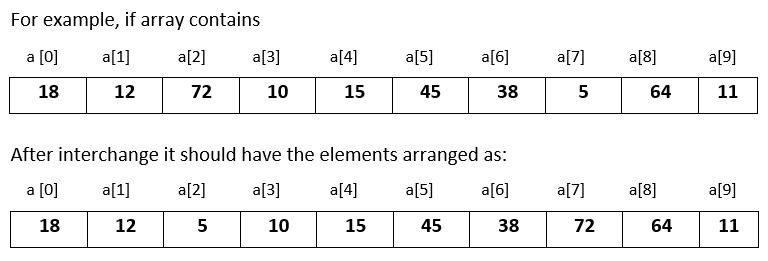# Input 10 numbers in integer array and swap the largest and smallest number in Python

###### Arrays - Question 6

In this question, we will see how to input 10 numbers in integer array and interchange the largest number with the smallest number within the array and print the modified array in Python programming. To know more about arrays click on the arrays lesson.

Q6) Write a program in Python to input 10 numbers in integer array and interchange the largest number with the smallest number within the array and print the modified array. Assume that there is only one largest and smallest number.#### Program

``````from array import *

ln=0; lnp=0; sn=0; snp=0
a=array('i',[])

print('Enter 10 numbers')
for i in range(10):
a.append(int(input()))

for i in range(10):
if i==0:
ln=a[i]
sn=a[i]
lnp=i
snp=i

elif a[i]>ln:
ln=a[i]
lnp=i

elif a[i]<sn:
sn=a[i]
snp=i

a[lnp]=sn
a[snp]=ln

print('\nArray after interchanging largest with the smallest');
for n in a:
print(n,end=' ')``````

#### Output

```Enter 10 numbers
18
12
72
10
15
45
38
5
64
11

Array after interchanging largest with the smallest
18 12 5 10 15 45 38 72 64 11```